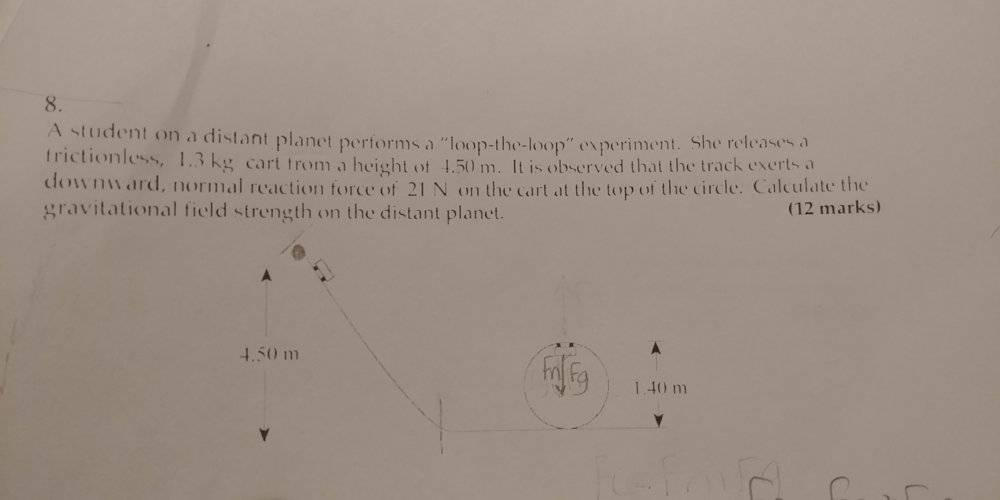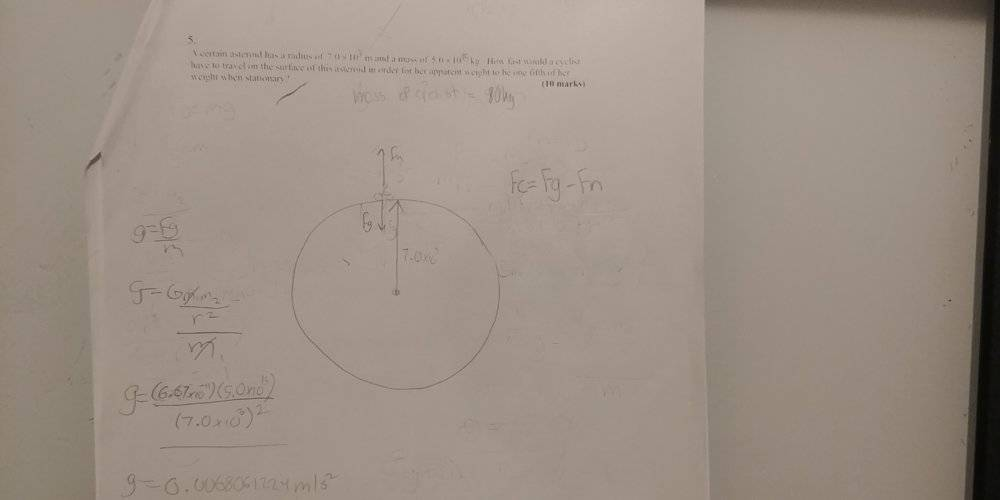# [Grade 12 Physics] Circular/gravitational motion

Homework Statement:
Question 1. A student on a distant planet performs a "loop-the-loop" experiment. She releases a frictionless 1.3kg cart from a height of 4.50m. It is observed that the track exerts a downward, normal reaction force of 21 N on the cart at the top of the circle. Calculate the gravitational field strength of the distant planet.

Question 2.
A certain asteroid has a radius of 7.0x10^3 m and a mass of 5.0x10^15 kg. How fast would a cyclist have to travel on the surface of this asteroid in order for her apparent weight to be one-fifth of her weight when stationary.
Relevant Equations:
Fcentripetal = ma
a = v^2 / r

Fg = GMm / r^2

Potential Energy = -GMm / r
Kinetic Energy = 0.5(mV^2)
Total Energy (Ek + Ep) = -0.5(GMm) / r

g = Fg / m
Diagram for question 1:I know the mass, I need Fg.

My work:

Main equation: g = Fg/m I need to find Fg.

1. Fg= Fc - Fn [Fn = 21 N Fc = ?] {I need to find Fc.}
2. Fc = ma --> Fc = (mV^2)/ r [Mass = 1.3kg V = ? r = 0.70] {Now I need the velocity at that point where Fn = 21 N (the top of the loop-the-loop)}
3. I break up the course into parts. I use the conservation of energy to try and solve the first part (4.50m) and use that velocity to solve the second parts velocity at the top (the loop-the-loop).
NOTE: I also see that there is a flat surface between the hill and the loop-the-loop but it's a frictionless cart so that wouldn't matter right?

Epi = Ekf --> -GMm/r = 1/2(mV^2) <---- PROBLEM. When I solve this I can't square root it since it's a negative.

Let's say I solved this and found the velocity. For the "loop-the-loop", would the equation for the conservation of energy be Eki = Epf + Ekf?

Diagram for question 2:So I started out by trying to find the strength of the gravitational field as I wanted to use Fc = Fg - Fn to find the velocity but I didn't have the mass of the cyclist. I tried to cross out all the masses on the equation but Fn prevented me from doing that. I don't know what I can do here.

## Answers and Replies

etotheipi
Epi = Ekf --> -GMm/r = 1/2(mV^2) <---- PROBLEM. When I solve this I can't square root it since it's a negative.

You're mixing and matching expressions with different reference points for gravitational potential energy. It is certainly correct that for the planet-rollercoaster system, between the top of the ramp and the top of the loop,

##\text{GPE}_1 + \text{KE}_1 = \text{GPE}_2 + \text{KE}_2##

##\text{KE}_1## is obviously 0. Now the more common approach is to use the approximation ##\text{GPE} = mgh## with the zero reference point set somewhere convenient, usually the surface of the planet. Here, ##\text{GPE}_1 = mgh_1## and ##\text{GPE}_2 = mgh_2##.

You've used ##\text{GPE} = -\frac{GMm}{r}##, which is the exact expression. It's correct, but note that you'd need to factor in the mass and radius of the planet (is that possible here...?). In this case, ##\text{GPE}_1 = -\frac{GMm}{r_1}## and ##\text{GPE}_2 = -\frac{GMm}{r_2}##. The final ##\text{GPE}## is not zero, as you have written, since the zero reference is at infinity!

Last edited by a moderator:
So GPE1 = GPE2 + KE2 ?

etotheipi
So GPE1 = GPE2 + KE2 ?

You bet

But what would the height value for the GPE2 be? Like the r value

Oh wait, I was thinking of the law of conservation of energy wrong. I think I get it now. On one of my other questions, there was a force of friction but this problem is frictionless. So, the GPE1 is for the whole system. thanks :)

Could you also help me with Question 2. ?

etotheipi
Could you also help me with Question 2. ?

Are you aware of how centrifugal forces arise in rotating frames of reference?

haruspex
Science Advisor
Homework Helper
Gold Member
2020 Award
So I started out by trying to find the strength of the gravitational field as I wanted to use Fc = Fg - Fn to find the velocity but I didn't have the mass of the cyclist. I tried to cross out all the masses on the equation but Fn prevented me from doing that. I don't know what I can do here.
Just put in her mass as m. Only get worried if it hasn’t cancelled by the end.

•etotheipi
I tried that but I'll see if I made a mistake, I probably did. For the other question, do you get 16.2m/s^2 for the gravitational strength? I'm just checking because it seems very big.

haruspex
Science Advisor
Homework Helper
Gold Member
2020 Award
I tried that but I'll see if I made a mistake, I probably did. For the other question, do you get 16.2m/s^2 for the gravitational strength? I'm just checking because it seems very big.
Yes, it should be rather smaller. If you can't find your mistake, please post your working.

EP1 = EP2 + EK2
((-6.67x10^-11)(1.3) / 4.50) = ((-6.67x10^-11)(1.3) / 1.40) + 1/2(v^2)

V = 9.237621478x10^-6

Fc = (1.3)(9.237621478x10^-6) / 0.70

Fc = 1.715558277x10^-5

Fc - 21 = Fg

1.715558277x10^-5 - 21 = Fg

Fg = 16.2m/s^2

haruspex
Science Advisor
Homework Helper
Gold Member
2020 Award
((-6.67x10^-11)(1.3) / 4.50)
That appears to be Gm/r. That is not an energy. It is a gravitational potential, but it would be for the gravitational field generated by the mass of the cyclist, as felt at the ground.

Please don't plug in numbers straight away. Keep everything symbolic until the end. That's less typing and calculation for you, and much easier to read for everyone else. Call the cyclist's mass m, the loop radius r, and the starting height h.

You are trying to find local g, and you can assume it is reasonably constant. So think of it as being on earth, just with weaker gravity.
What potential energy is lost in reaching the top of the loop?

How am I supposed to calculate the loss of potential energy when I don't have the mass of the planet or the gravitational field strength? On the equation above, I cancelled out the mass of the planet but I didn't know if that worked.

haruspex
Science Advisor
Homework Helper
Gold Member
2020 Award
How am I supposed to calculate the loss of potential energy when I don't have the mass of the planet or the gravitational field strength? On the equation above, I cancelled out the mass of the planet but I didn't know if that worked.
just call the gravitational acceleration g.

so the I found that the potential energy lost was 4.03g's. Using the law of conservation of energy, that should equal the kinetic energy:

4.03g = 1/2(mv^2)

which turned out to be: 6.2g = v^2 (I'm not going to square root this because we need v^2 in the next equation)

mV^2 / r --> (1.3)(6.2g) / 0.70

this turns out to be: 8.06g/0.70 - 21 = (1.3)(g)

I solve for g and get 2.06m/s^s. Is this correct?

Last edited:
haruspex
Science Advisor
Homework Helper
Gold Member
2020 Award
4.03g =
I asked you not to plug in numbers straightaway.
Starting height is h, loop radius r, mass m, gravity g.
Keep it symbolic.

EP1 = EP2 + EK2
mgh - mg2r = 1/2(mV^2)

mgh - mg2r = Change in EP
Change in EP = 4.03g
Change in EP = Kinetic energy
4.03g = 1/2(mv^2)

Last edited:
haruspex
Science Advisor
Homework Helper
Gold Member
2020 Award
mgh - mg2r = 1/2(mV^2)
Good. But don't then find the numeric value; that is wasted effort and makes it harder to follow your reasoning.

But I just realised there is some confusion here. You posted two questions in one thread (which the guidelines say not to do).
I seem to have reverted to the first question. Did you finish the first question satisfactorily, and now we are on the second?

I think I finished the first question, I got 2.06 m/s^2 for my gravitation strength and was wondering if you could confirm this.

haruspex
Science Advisor
Homework Helper
Gold Member
2020 Award
I think I finished the first question, I got 2.06 m/s^2 for my gravitation strength and was wondering if you could confirm this.
Yes, that's the right answer.
To illustrate symbolic working:
##\frac 12mv^2=mg(h-2r)##
##F+mg=m\frac{v^2}r=2mg(\frac hr-2)##
##F=mg(2\frac hr-5)##
##g=\frac F{m(2\frac hr-5)}##
Now you can plug in numbers.

I'll comment on problem 2 in next post.

haruspex
Science Advisor
Homework Helper
Gold Member
2020 Award
For question 2, once again, define symbols for all the variables.
Planet mass = M, radius R, cyclist mass = m, planet gravity = g, cyclist speed = v.
Write equations for actual weight and apparent weight.